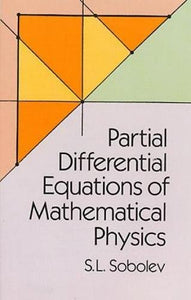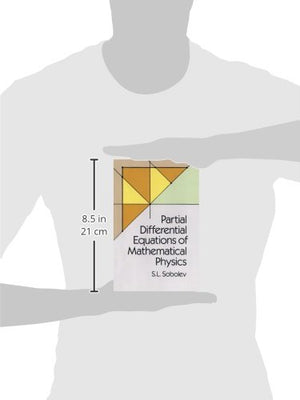# Partial Differential Equations of Mathematical Physics (Dover Books on Physics)

• Publish Date: 2011-02-17
• Binding: Paperback
• Author: S. L. Sobolev;Physics
Regular price \$43.73 Sale price \$14.73

The classical partial differential equations of mathematical physics, formulated by the great mathematicians of the 19th century, remain today the basis of investigation into waves, heat conduction, hydrodynamics, and other physical problems.
In this comprehensive treatment by a well-known Soviet mathematician, the equations are presented and explained in a manner especially designed to be accessible to the novice in the field. The reader is assumed to have no previous knowledge other than elementary analysis. From there, more advanced concepts are developed in detail and with great precision; moreover, theorems are often approached through the study of special simpler cases, before being proved in their full generality, and are applied to many particular physical problems.
After deriving the fundamental equations, the author provides illuminating expositions of such topics as Riemann's method, Lebesgue integration of multiple integrals, the equation of heat conduction, Laplace's equation and Poisson's equation, the theory of integral equations, Green's function, Fourier's method, harmonic polynomials and spherical functions, and much more.
For this third edition, various improvements in style and clarifications of the presentations were made, including a simplification of the theory of multiple Lebesgue integrals and greater precision in the proof of the Fourier method. Finally, the translation is both idiomatic as well as accurate, making the vast amount of information in this book more readily accessible to the English reader.